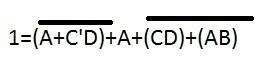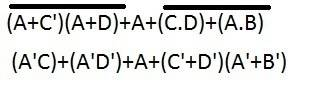# Boolean Algebra prove

## Homework StatementBoolean Algebra

## The Attempt at a Solution

I use the distri to change the A+C'.D and Demorgan.
Should i use dis and Demorgan firstly like below?Which property I should use in the next step?
If the first part is wrong,which property i should use first?
Sorry for the small pictures!
Thanks

jedishrfu
Mentor
Continue to use demorgan until you get down into the simplest form then apply algebra reduction rules.

So are you trying to prove:

(A + C'D)' + A + (CD + AB)' = 1

Just want to make sure we understand the problem as the bar above terms in your images looks like it might include the A term.

Last edited:
Continue to use demorgan until you get down into the simplest form then apply algebra reduction rules.

So are you trying to prove:

(A + C'D)' + A + (CD + AB)' = 1

Just want to make sure we understand the problem as the bar above terms in your images looks like it might include the A term.

Sorry for the vague picture.The bar not include in the A term.
Should i use the Demorgan in the whole question firstly including the A.
As the picture shows,then,i continue to use Distributive or Absorption,but failed.

jedishrfu
Mentor
Sorry for the vague picture.The bar not include in the A term.
Should i use the Demorgan in the whole question firstly including the A.
As the picture shows,then,i continue to use Distributive or Absorption,but failed.

In your final expression do you have any terms like this?

X + X' + ...... =?= 1

In your final expression do you have any terms like this?

X + X' + ...... =?= 1

I continue to calculate following the picture show on the The attempt at a solution

First:
A'(C+D')+(A+C'+D')(A+A'+B') Use distributive
A'(C+D')+(A+C'+D')(1+B')
But i was wondering whether i can change to 1+B' to 1,because the list only show that 1+A=1

Second:
A'(C+D')+A+A'C'+B'C'+A'D'+B'D'
A'(C+D')+A+A'(C'+D')+B'(C'+D')

jedishrfu
Mentor
It seems you are almost there. The last expression

A'(C+D')+A+A'(C'+D')+B'(C'+D')

can be expanded into

A'C + A'D' + A + A'C' + A'D' + B'C' + B'D'

and then combine some terms to get:

A'(C + C') + A + A'D' + B'C' + B' D'

Do you see how to finish it?

HINT: remember that X' + X = 1 and that 1+ANYTHING = 1 in boolean algebra

it seems you are almost there. The last expression

a'(c+d')+a+a'(c'+d')+b'(c'+d')

can be expanded into

a'c + a'd' + a + a'c' + a'd' + b'c' + b'd'

and then combine some terms to get:

A'(c + c') + a + a'd' + b'c' + b' d'

do you see how to finish it?

Hint: Remember that x' + x = 1 and that 1+anything = 1 in boolean algebra

a'+ a + a'd' + b'c' + b' d'
1+a'd' + b'c' + b' d'
1

jedishrfu
Mentor
a'+ a + a'd' + b'c' + b' d'
1+a'd' + b'c' + b' d'
1

Thats great!

It floored me too when I got to the second to last step and thought how am I going to reduce that and then I saw the A + A' terms
and realized the other terms just didn't matter.

•1 person
Thats great!

It floored me too when I got to the second to last step and thought how am I going to reduce that and then I saw the A + A' terms
and realized the other terms just didn't matter.

Thanks you!!!